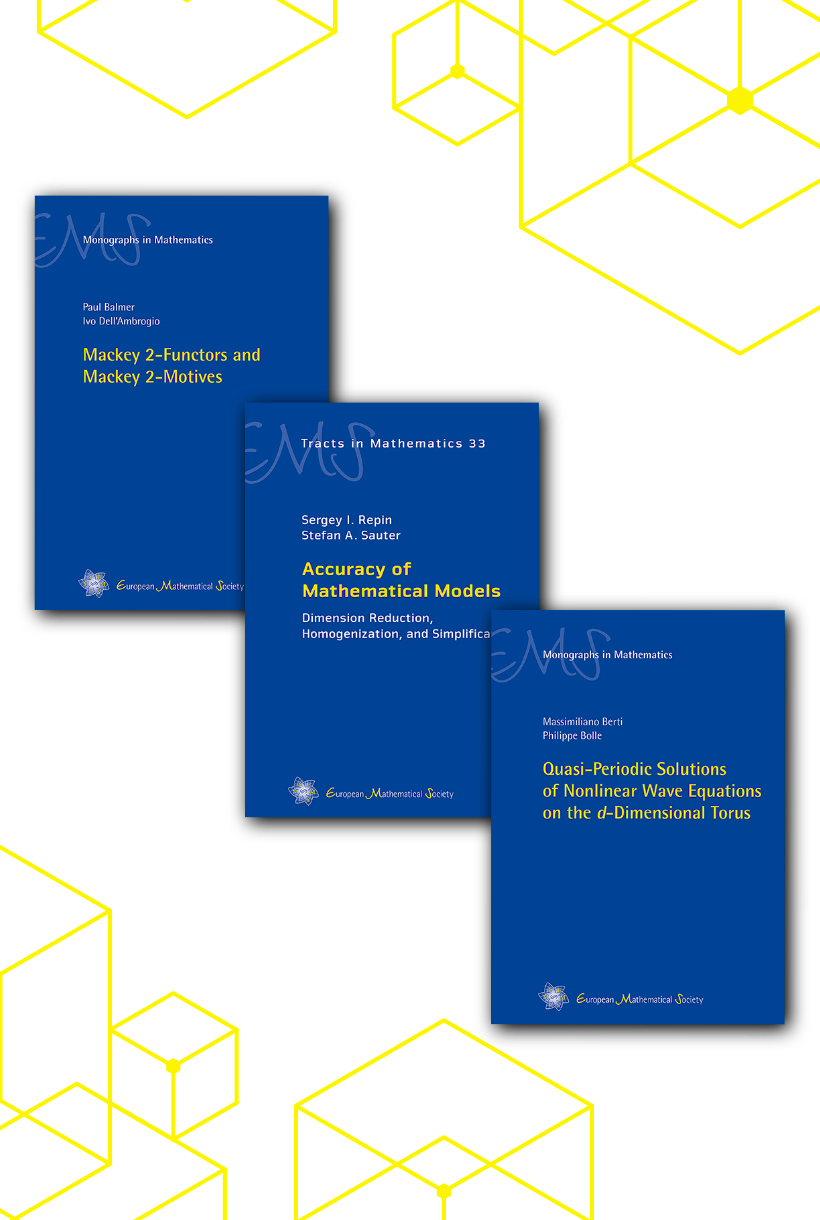21 September 2020

# New Book Releases – Autumn 2020

Apostolos Damialis

An overview of our new book releases in autumn 2020.Expanding our book publications output in 2020, these are our latest book titles:

• Mackey 2-Functors and Mackey 2-Motives

• Accuracy of Mathematical Models

• Quasi-Periodic Solutions of Nonlinear Wave Equations on the d-Dimensional Torus

Read on to find out more about each title.

#### Mackey 2-Functors and Mackey 2-Motives (August 2020)

Paul Balmer (University of California, Los Angeles, USA) Ivo Dell'Ambrogio (Université de Lille, France)

This book is dedicated to equivariant mathematics, specifically the study of additive categories of objects with actions of finite groups. The framework of Mackey 2-functors axiomatizes the variance of such categories as a function of the group. In other words, it provides a categorification of the widely used notion of Mackey functor, familiar to representation theorists and topologists.

The book contains an extended catalogue of examples of such Mackey 2-functors that are already in use in many mathematical fields from algebra to topology, from geometry to KK-theory. Among the first results of the theory, the ambidexterity theorem gives a way to construct further examples and the separable monadicity theorem explains how the value of a Mackey 2-functor at a subgroup can be carved out of the value at a larger group, by a construction that generalizes ordinary localization in the same way that the étale topology generalizes the Zariski topology. The second part of the book provides a motivic approach to Mackey 2-functors, 2-categorifying the well-known span construction of Dress and Lindner. This motivic theory culminates with the following application: The idempotents of Yoshida’s crossed Burnside ring are the universal source of block decompositions.

The book is self-contained, with appendices providing extensive background and terminology. It is written for graduate students and more advanced researchers interested in category theory, representation theory and topology.

#### Accuracy of Mathematical Models(August 2020)

Series: EMS Tracts in Mathematics

Sergey I. Repin (Russian Academy of Sciences, St. Petersburg, Russian Federation) Stefan A. Sauter (Universität Zürich, Switzerland)

The expansion of scientific knowledge and the development of technology are strongly connected with quantitative analysis of mathematical models. Accuracy and reliability are the key properties we wish to understand and control.

This book presents a unified approach to the analysis of accuracy of deterministic mathematical models described by variational problems and partial differential equations of elliptic type. It is based on new mathematical methods developed to estimate the distance between a solution of a boundary value problem and any function in the admissible functional class associated with the problem in question. The theory is presented for a wide class of elliptic variational problems. It is applied to the investigation of modelling errors arising in dimension reduction, homogenization, simplification, and various conversion methods (penalization, linearization, regularization, etc.). A collection of examples illustrates the performance of error estimates.

#### Quasi-Periodic Solutions of Nonlinear Wave Equations on the d-Dimensional Torus (October 2020)

Massimiliano Berti (SISSA, Trieste, Italy) Philippe Bolle (Avignon Université, France)

Many partial differential equations (PDEs) arising in physics, such as the nonlinear wave equation and the Schrödinger equation, can be viewed as infinite-dimensional Hamiltonian systems. In the last thirty years, several existence results of time quasi-periodic solutions have been proved adopting a "dynamical systems" point of view. Most of them deal with equations in one space dimension, whereas for multidimensional PDEs a satisfactory picture is still under construction.

An updated introduction to the now rich subject of KAM theory for PDEs is provided in the first part of this research monograph. We then focus on the nonlinear wave equation, endowed with periodic boundary conditions. The main result of the monograph proves the bifurcation of small amplitude finite-dimensional invariant tori for this equation, in any space dimension. This is a difficult small divisor problem due to complex resonance phenomena between the normal mode frequencies of oscillations. The proof requires various mathematical methods, ranging from Nash–Moser and KAM theory to reduction techniques in Hamiltonian dynamics and multiscale analysis for quasi-periodic linear operators, which are presented in a systematic and self-contained way. Some of the techniques introduced in this monograph have deep connections with those used in Anderson localization theory.

This book will be useful to researchers who are interested in small divisor problems, particularly in the setting of Hamiltonian PDEs, and who wish to get acquainted with recent developments in the field.